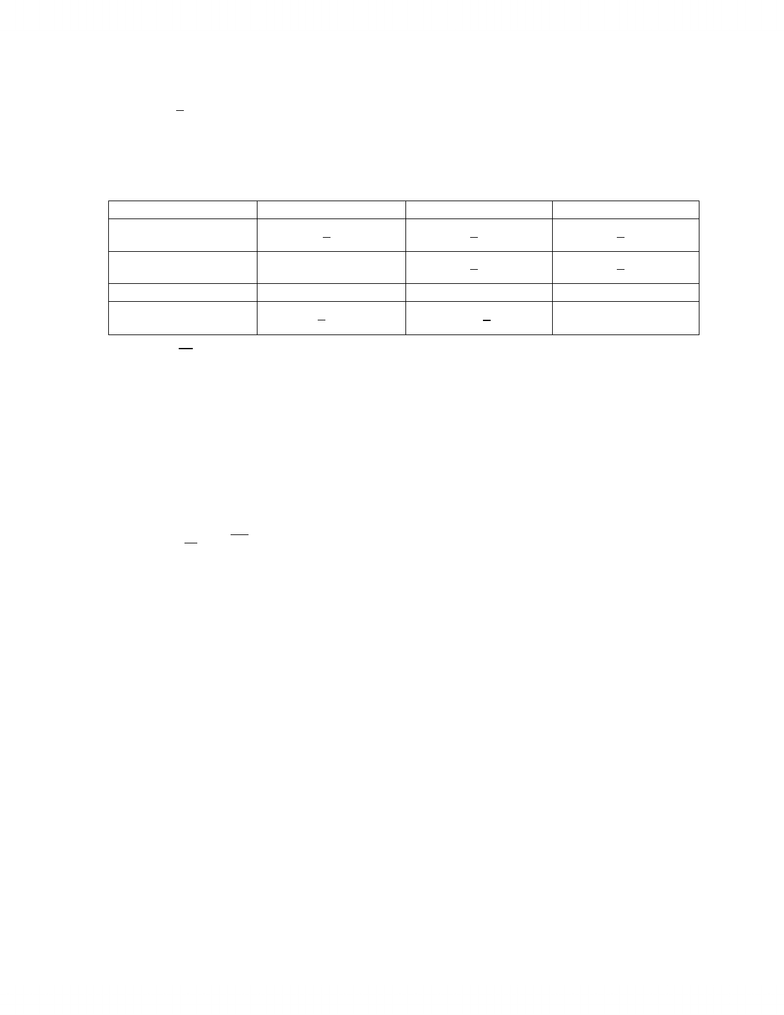Study Guides (390,000)
CA (150,000)
UTSG (10,000)
CHM (100)
Midterm

# CHM220H1 Study Guide - Midterm Guide: Irreversible Process, Bond-Dissociation Energy, Endergonic Reaction

Department
Chemistry
Course Code
CHM220H1
Professor
Douglas Mc Intosh
Study Guide
Midterm

This preview shows page 1. to view the full 5 pages of the document.CHM220 Midterm Study Sheet
P a g e | 1
1 Definitions
System is the reaction, surroundings is everything else; together is the universe
System:
o Open mass and energy exchange
o Closed only energy exchange
o Isolated no mass nor energy exchange
o Diathermic allows energy to leave system as heat
o Adiabatic means no heat exchange between system and surroundings
Properties of Matter
o Extensive properties values directly proportional to amount of material
o Intensive properties values do not depend on amount of material
o Intensive properties usually defined as ratio of two extensive properties
State function is path independent
Absolute zero is -273.15C
Boyle’s Law
Charles’ Law
Ideal Gas Equation



Ideal gas occupies 22.414L at 1atm and 273.15K (STP)
o Assuming gas molecules possess negligible volume
o No interaction between molecules
Gasses have attracted and repulsive forces
o Idea gas only useful for high T and low P
Z= compressibility factor = PV/nRT
o Z is 1 for idea gas at any P or T
Van der Waals


o a for attractive intermolecular forces
o b for finite volume of molecules
o Also unreliable at high P and low T
o Slight correlation between a and boiling point
3 Molecules and their Energies
Average kinetic energy for one molecule:

Average kinetic energy for one mole of gas:

Unlock to view full version

Only page 1 are available for preview. Some parts have been intentionally blurred.CHM220 Midterm Study Sheet
P a g e | 2


Internal energy is U
Etot = ETrans + ERot + EVib + EElec
Where N is the number of bonds in the molecule
Monoatomic
Linear
Non-linear
Translational



Roatational
0


Vibrational
0


Total





; so remove T from total internal energy
Quantum Mechanics
o Different molecular energy level differences associated with each motion
10-17J for electronic
10-20J for vibrational
10-23J for rotational
10-37J for translational
o Easier to promote to higher energy level when difference is smaller
o Ratio of populations of molecules in two energy levels given by Boltzmann Distribution:

; N2/N1 < 1
o If E is large compared to kBT then molecules will crowd to lower energy levels
o Energy not quantized for translational motion
o Energy is quantized in rotational motion
o Most molecules in lower energy level at low temperatures for vibrational motion
o Almost all molecules in lower energy levels at low temperatures for electronic motion
o So that at room temperature, only translational and rotation motions contribute to U
and heat capacity
Heat Capacities
o C=U/T = q/T; q is heat required to raise T by T
o Cv=U/T = qV/T
o CP=H/T = qp/T
o Heat capacity equations only true for below 300K
o CP=a+BT; a and B are constants for given substance
o Cp>Cv since more work is done in constant pressure
Cp-Cv=R
o Cp=Cv for liquids and solids due to small change in volume
Heat capacities of liquids is greater than gases due to weak interactions
between molecules